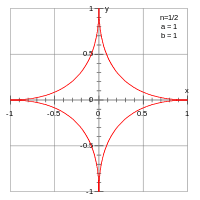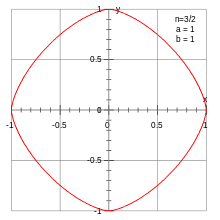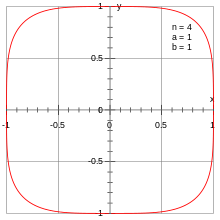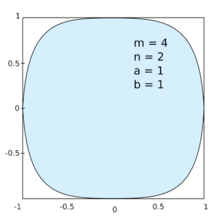Superellipse

A superellipse, also known as a Lamé curve after Gabriel Lamé, is a closed curve resembling the ellipse, retaining the geometric features of semi-major axis and semi-minor axis, and symmetry about them, but a different overall shape.

In the Cartesian coordinate system, the set of all points (x, y) on the curve satisfy the equationwhere n, a and b are positive numbers, and the vertical bars | | around a number indicate the absolute value of the number.

Specific cases

This formula defines a closed curve contained in the rectangle ax  +a and b  y  +b. The parameters a and b are called the semi-diameters of the curve.The superellipse looks like a four-armed star with concave (inwards-curved) sides. For n = 1/2, in particular, each of the four arcs is a segment of a parabola.The superellipse with n =  1⁄2, a = b = 1The curve is a rhombus with corners (±a, 0) and (0, ±b).The curve looks like a rhombus with those same corners but with convex (outwards-curved) sides. The curvature increases without limit as one approaches its extreme points.The superellipse with n =  3⁄2, a = b = 1The curve is an ordinary ellipse (in particular, a circle if a = b).The curve looks superficially like a rectangle with rounded corners. The curvature is zero at the points (±a, 0) and (0, ±b).Squircle, the superellipse with n = 4, a = b = 1

If n < 2, the figure is also called a hypoellipse; if n > 2, a hyperellipse.

When n  1 and a = b, the superellipse is the boundary of a ball of R2 in the n-norm.

The extreme points of the superellipse are (±a, 0) and (0, ±b), and its four "corners" are (±sa, ±sb), where(sometimes called the "superness").

Mathematical properties

When n is a nonzero rational number p/q (in lowest terms), then each quadrant of the superellipse is a plane algebraic curve. For positive n the order is pq; for negative n the order is 2pq. In particular, when a = b = 1 and n is an even integer, then it is a Fermat curve of degree n. In that case it is non-singular, but in general it will be singular. If the numerator is not even, then the curve is pasted together from portions of the same algebraic curve in different orientations.

The curve is given by the parametric equationsorwhere the sign function isThe area inside the superellipse can be expressed in terms of the gamma function, Γ(x), asThe pedal curve is relatively straightforward to compute. Specifically, the pedal ofis given in polar coordinates byGeneralizationsExample of the generalized superellipse with m  n.

The superellipse is further generalized as:or(Note thatis not a physical angle of the figure, but just a parameter.)

History

The general Cartesian notation of the form comes from the French mathematician Gabriel Lamé (17951870), who generalized the equation for the ellipse.The outer outlines of the letters 'o' and 'O' in Zapf's Melior typeface are described by superellipses with n = log(1/2) / log (7/9) ≈ 2.758

Hermann Zapf's typeface Melior, published in 1952, uses superellipses for letters such as o. Thirty years later Donald Knuth would build the ability to choose between true ellipses and superellipses (both approximated by cubic splines) into his Computer Modern type family.

The superellipse was named by the Danish poet and scientist Piet Hein (1905–1996) though he did not discover it as it is sometimes claimed. In 1959, city planners in Stockholm, Sweden announced a design challenge for a roundabout in their city square Sergels Torg. Piet Hein's winning proposal was based on a superellipse with n = 2.5 and a/b = 6/5. As he explained it:

Man is the animal that draws lines which he himself then stumbles over. In the whole pattern of civilization there have been two tendencies, one toward straight lines and rectangular patterns and one toward circular lines. There are reasons, mechanical and psychological, for both tendencies. Things made with straight lines fit well together and save space. And we can move easily physically or mentally around things made with round lines. But we are in a straitjacket, having to accept one or the other, when often some intermediate form would be better. To draw something freehand such as the patchwork traffic circle they tried in Stockholm will not do. It isn't fixed, isn't definite like a circle or square. You don't know what it is. It isn't esthetically satisfying. The super-ellipse solved the problem. It is neither round nor rectangular, but in between. Yet it is fixed, it is definite it has a unity.

Sergels Torg was completed in 1967. Meanwhile, Piet Hein went on to use the superellipse in other artifacts, such as beds, dishes, tables, etc. By rotating a superellipse around the longest axis, he created the superegg, a solid egg-like shape that could stand upright on a flat surface, and was marketed as a novelty toy.

In 1968, when negotiators in Paris for the Vietnam War could not agree on the shape of the negotiating table, Balinski, Kieron Underwood and Holt suggested a superelliptical table in a letter to the New York Times. The superellipse was used for the shape of the 1968 Azteca Olympic Stadium, in Mexico City.

Waldo R. Tobler developed a map projection, the Tobler hyperelliptical projection, published in 1973, in which the meridians are arcs of superellipses.

Three connected superellipses are used in the logo of the Pittsburgh Steelers.

In computing, mobile operating system iOS uses a superellipse curve for app icons, replacing the rounded corners style used up to version 6.Wikimedia Commons has media related to Superellipse.